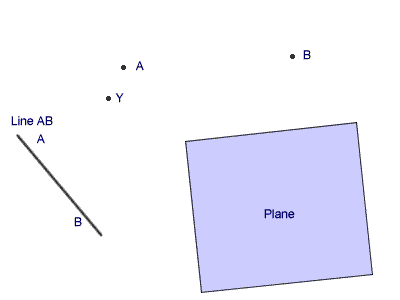# Terms You Need To Know

#### Point:

A point shows location and has no size or dimension. A point is represented by a dot and is usually named with a letter of choice. In the graphic below, the points are labelled by a nearby letter.

#### Line:

A line is a set of points extends in two opposite directions without end. A line is one-dimensional and has no width. It is identified by naming two points on the line or by writing a lowercase letter of choice after the line.

The notation, for example, AB (written with a line symbol <---> over the letters), is read as "line AB" and refers to the line that has points A and B.

#### Plane:

A plane is a flat surface that has no thickness and extends without ending in ALL directions. It is a two-dimensional object. A plane is represented by a parallelogram and may be named by writing an uppercase letter of choice in one of its corners. I will go into detail about what a parallelogram is in future lessons. For now, think of a parallelogram as a "window pane."For simplicity, you might want to think of a plane as an infinitely large sheet of paper.

The diagram below shows three points, a line, and a plane.#### Line Segment:

A line line segment is part of a line having two points, called endpoints. It also has points between the endpoints. A line segment does not have a set of CONTINUOUS points like a line does. Endpoint means that a line has a beginning and an end. The notation for a line segment in a bar over any letter of choice. Say AB has a bar over it, you would read it as "line segment AB."

#### Ray:

A ray is part of a line having one endpoint and a set of all points on one side of the endpoint. A ray is always named by using two letters of choice. The first letter must be the endpoint. We draw an arrow with an endpoint over the letters.

#### Angle:

An angle is the union of two rays having the same endpoint. The endpoint of an angle is called the vertex; the rays are called the sides of the angle.There are a few additional terms in geometry that need to be understood as well. They define relationships between geometric objects:

Collinear Points: points that lie on the same line.

Coplanar Points: points that lie in the same plane.

Opposite Rays: 2 rays that lie on the same line, with a common endpoint and no other points in common. Opposite rays form a straight line and/or a straight angle that equals 180º.

Parallel Lines: two coplanar lines that do not intersect.